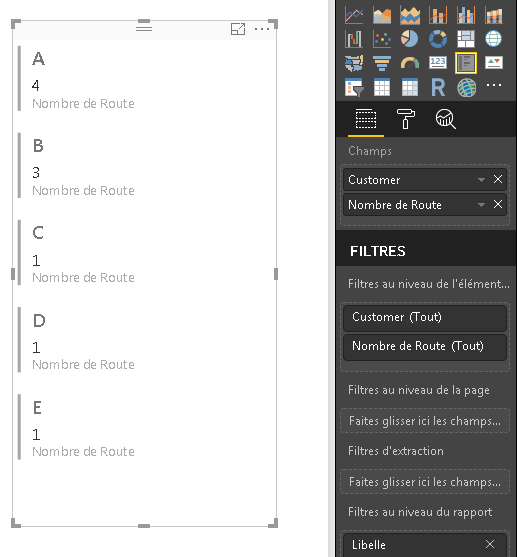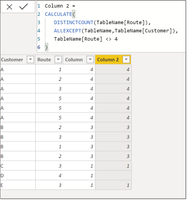cancel
Showing results for
Did you mean:Helper I

## Count based on another column

Hi PBI Community,

I am trying to solve the following issue. I want to count how many different routes does a Customer follow. In other words, a distinct count of the column "Route" per Customer.

Is this possible to do in a calculated column?

Below is the table and on the right is the desired Count column.2 ACCEPTED SOLUTIONSSuper User

hello @misul,

You could try:

```Column =
CALCULATE(
DISTINCTCOUNT(Table1[Route]),
ALLEXCEPT(Table1,Table1[Customer])
)```

Did my answers help arrive at a solution? Give it a kudos by clicking the Thumbs Up!

Proud to be a Super User!Helper I

@ChrisMendoza Correct, the intention is to use DistinctCountofRoutes as a slicer.

When I use the calculated column, the values are summed. So I put the column to "Don't Summarize" and it seems to work now. Thanks!

Calculated Column:

```Column =
CALCULATE(
DISTINCTCOUNT(Table1[Route]),
ALLEXCEPT(Table1,Table1[Customer])
)```

Measure:

```Measure=
CALCULATE(
DISTINCTCOUNT(Table1[Route]),
ALLEXCEPT(Table1,Table1[Customer])
)```

I realised that the same formula can be used as a measure too. But as measures cannot be used in Slicers. I will stick with Calculated Column (without summarizing it).

17 REPLIES 17Anonymous
Not applicable

iSolution Sage

@misul

I've found something usefull if you want to display it in a cardbox : just put both of your column in a multiple line card box and select the distinct optionHelper I

@quentin_vigne

Thanks for your help. But I need the Distinct Count as a Column/Measure so that I can use this as a slicer later on.

When I select Distinct Count = 1, show me all the customers which are having 1 route (i.e Customer C,D,E)

When I select Distinct Coutn =4, show me all the customers which are having 4 Routes. (i.e. Customer A)

Any further ideas?Super User

hello @misul,

You could try:

```Column =
CALCULATE(
DISTINCTCOUNT(Table1[Route]),
ALLEXCEPT(Table1,Table1[Customer])
)```

Did my answers help arrive at a solution? Give it a kudos by clicking the Thumbs Up!

Proud to be a Super User!Frequent Visitor

Thank you so much for the solution.Frequent Visitor

Hi,

This is great. I also tried to use this formula in my dashboard, but unfortunately this didn't helped me much. The problem starts when I apply a filter to other columns.

For e.g. in the given example, Customer A has 4 routes. Let's assume there are other 3 customers - X, Y & Z who also have 4 unique routes. Now if I have a column for region (say EU, APAC, LATAM, etc.) and if I select 1 region, let's say EU, so customer A who has 2 unique routes in EU, should only show 2. But with this formula it still shows 4.Anonymous
Not applicable

@ChrisMendoza , In this example, if I need to filter the Table1[Route] <> 4, for example, How I can change the formula to Calculate DistinctCount Table1[Route] by Table1[Customer]) but not considering the records with Table1[Route] = 4.

I tried to include the filter in the table but I got the message "Parameter is not the correct type"

In my case mey formula is:

CALCULATE(DISTINCTCOUNT(Suppliers[PART_STATUS]),
ALLEXCEPT( FILTER(Suppliers, RELATED(Suppliers[PART_STATUS])<> "U") ,
Suppliers[KEY]) ).

thank you!Super User

@Anonymous -
I suppose it depends on what you're trying to accomplish. Reviewing the OP, their need seems different than yours. A calculated column has purpose as well as a measure having it's own purpose.

``````Column 2 =
CALCULATE(
DISTINCTCOUNT(TableName[Route]),
ALLEXCEPT(TableName,TableName[Customer]),
TableName[Route] <> 4
)``````

Produces:However when you represent it in a visual it likely doesn't produce the result you're looking for. I've included a measure so you can see the differences between [Column] / [Column 2] and a [Measure].``````Measure =
CALCULATE(
DISTINCTCOUNT(TableName[Route]),
ALLEXCEPT(TableName,TableName[Customer]),
TableName[Route] <> 4
)``````

Maybe you could post your question in the forum with your desired outcome if my response doesn't solve your issue?

Did my answers help arrive at a solution? Give it a kudos by clicking the Thumbs Up!

Proud to be a Super User!Anonymous
Not applicable

@ChrisMendoza , thank you very much! It worked using the formula suggested in a new column. Perfect!!! 🙂Helper I

@ChrisMendoza Thanks for your idea. This is working in my dummy file. But not in my database (which has much more records).

There are only 15 routes available. But the measure you gave is returning values like - 3000, 200 etc.

Any ideas?

Appreciated.Super User

Is it summing the column? I wouldn't imagine that you would actually use the calculated column for anything except for some math operation or a slicer.

Based off your response to someone else, your intention is to use a slicer to filter the data set, so the slicer is generating > ~15 routes which you did not expect?

Did my answers help arrive at a solution? Give it a kudos by clicking the Thumbs Up!

Proud to be a Super User!Helper I

@ChrisMendoza Correct, the intention is to use DistinctCountofRoutes as a slicer.

When I use the calculated column, the values are summed. So I put the column to "Don't Summarize" and it seems to work now. Thanks!

Calculated Column:

```Column =
CALCULATE(
DISTINCTCOUNT(Table1[Route]),
ALLEXCEPT(Table1,Table1[Customer])
)```

Measure:

```Measure=
CALCULATE(
DISTINCTCOUNT(Table1[Route]),
ALLEXCEPT(Table1,Table1[Customer])
)```

I realised that the same formula can be used as a measure too. But as measures cannot be used in Slicers. I will stick with Calculated Column (without summarizing it).Anonymous
Not applicable

Hello Dozer,

Since you are specifying it takes more time to a computer. Can you split the main table to have only "customer" and "route" and later join the new table by the customer and give appropriate direction?

Thanks,Frequent Visitor

Hi,

My solution:

Create a calculated column

```DistinctRoute by customer =
VAR CurrencyCustomar = 'Table'[Customar]
RETURN
CALCULATE(DISTINCTCOUNT('Table'[Route]); FILTER(ALL('Table');'Table'[Customar] = CurrencyCustomar))```Helper II

Wanted to thank you, your method worked perfect and I was unpivoting columns previously and wanted an easier way. Thank you!Helper I

@shapkovromanBI My database is very big, and this formula takes a very very long time to run. This isn't ideal..   formula is still being calculated, it has been a few minutes now.

Update after 10 minutes .. still running. This formula can't be used ..as it takes more than 10 minutes time to run on my database.

Any further ideas?Solution Sage

Hi @misul

What I would do is a global column :

`count = COUNTROWS(Table)/CALCULATE(COUNTROWS(Table);ALL(Table))`

And then you add both the customer column and the new count column to a Card Box

It will display Customer + Count of distinct